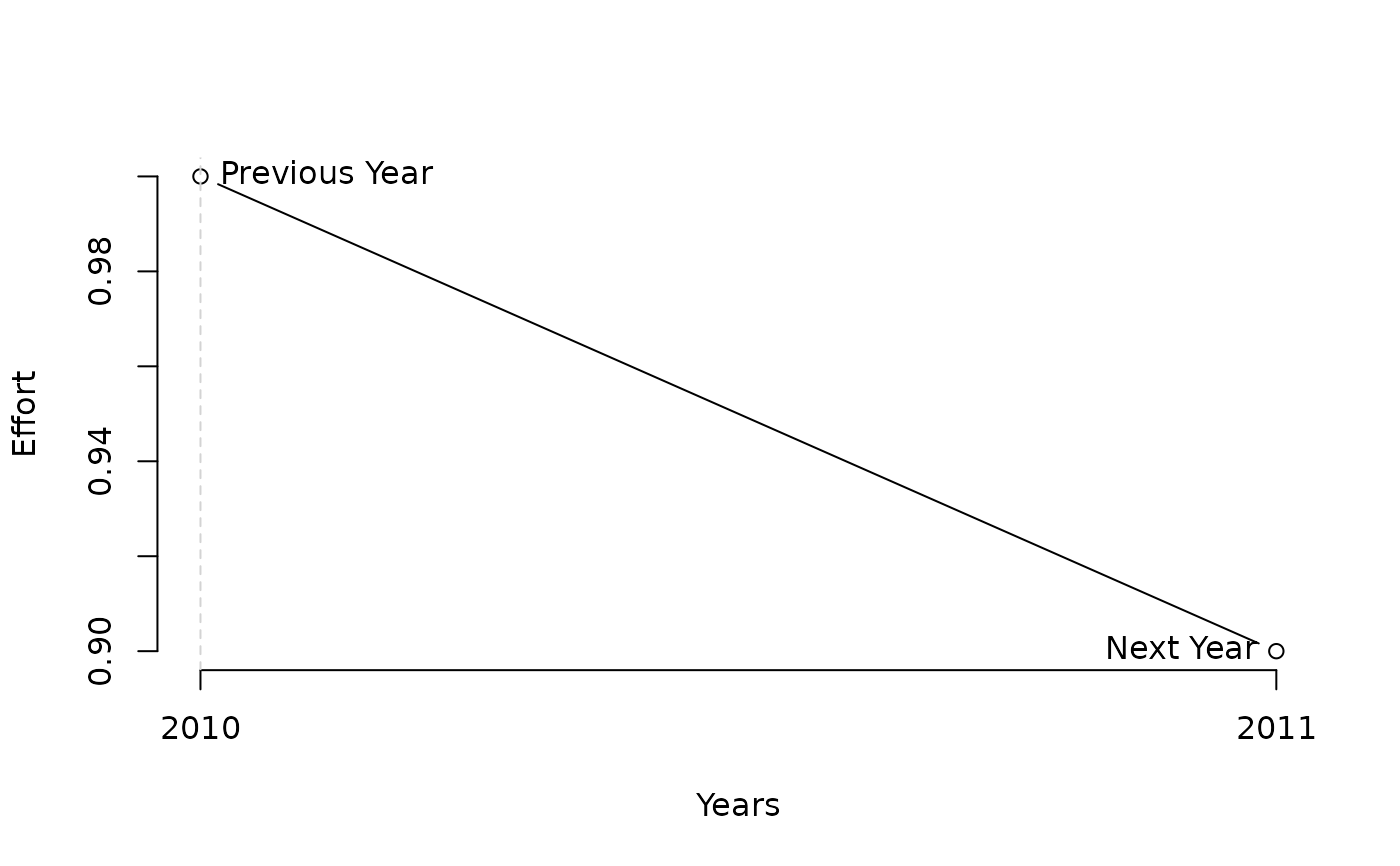Effort is adjusted using a simple rule that aims for a specified level of depletion.

DTe40(x, Data, reps = 100, plot = FALSE, alpha = 0.4, LB = 0.9, UB = 1.1)

DTe50(x, Data, reps = 100, plot = FALSE, alpha = 0.5, LB = 0.9, UB = 1.1)

## Arguments

x

A position in the data object

Data

A data object

reps

The number of stochastic samples of the MP recommendation(s)

plot

Logical. Show the plot?

alpha

The target level of depletion

LB

The lowest permitted factor of previous fishing effort

UB

The highest permitted factor of previous fishing effort

## Value

An object of class Rec-class with the TAE slot(s) populated

## Details

The TAE is calculated as: $$\textrm{TAE}_y = \frac{D}{\alpha} \textrm{TAE}_{y-1}$$ where $$D$$ is estimated current level of depletion and $$\alpha$$ is argument alpha specifying the target level of depletion.

The maximum fractional change in TAE is specified with arguments LB and UB

## Functions

• DTe40: Effort is adjusted to reach 40 percent stock depletion

• DTe50: Effort is adjusted to reach 50 percent stock depletion

## Required Data

See Data-class for information on the Data object

DTe40: Dep, MPeff

## Rendered Equations

See Online Documentation for correctly rendered equations

T. Carruthers

## Examples

DTe40(1, MSEtool::Atlantic_mackerel, plot=TRUE)#> Effort
#>    0.9